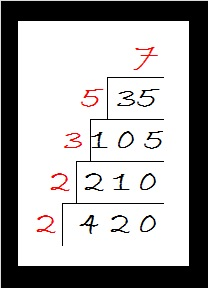# 420 Factor Trees

Did you know that the sum of all the prime numbers between 100 and 110 equals 420? Yes, 101 + 103 + 107 + 109 = 420.

Since 20 × 21 = 420, we know that 420 is the sum of the first 20 EVEN numbers. Thus,

• 2 + 4 + 6 + 8 + 10 + 12 + 14 + 16 + 18 + 20 + 22 + 24 + 26 + 28 + 30 + 32 + 34 + 36 + 38 + 40 = 420.

420 is the smallest number that can be divided evenly by all the natural numbers from 1 to 7.

420 has a LOT of factors, more than most people would think it does.  In fact, of all of the numbers from 1 to 420, there is only one number, 360, that has as many factors as 420 has.

There are 4 different prime numbers that can divide evenly into 420. Here are those factor trees:Many, but not all, of the factors of 420 are listed somewhere on those four trees.

• 420 is a composite number.
• Prime factorization: 420 = 2 x 2 x 3 x 5 x 7, which can be written 420 = (2^2) x 3 x 5 x 7
• The exponents in the prime factorization are 2, 1, 1, and 1. Adding one to each and multiplying we get (2 + 1)(1 + 1)(1 + 1)(1 + 1) = 3 x 2 x 2 x 2 = 24. Therefore 420 has exactly 24 factors.
• Factors of 420: 1, 2, 3, 4, 5, 6, 7, 10, 12, 14, 15, 20, 21, 28, 30, 35, 42, 60, 70, 84, 105, 140, 210, 420
• Factor pairs: 420 = 1 x 420, 2 x 210, 3 x 140, 4 x 105, 5 x 84, 6 x 70, 7 x 60, 10 x 42, 12 x 35, 14 x 30, 15 x 28, or 20 x 21
• Taking the factor pair with the largest square number factor, we get √420 = (√4)(√105) = 2√105 ≈ 20.4939Each factor pair, except 1 x 420, can make its own factor tree. Here are some factor trees featuring the other seven factor pairs:If I hadn’t made all the prime numbers in red, it’s possible that one or more of the prime numbers might get forgotten. That is why I prefer the cake method for finding the prime factorization of a number. All of the prime numbers are listed in numerical order on the outside of the cake.1.HM
•ivasallay